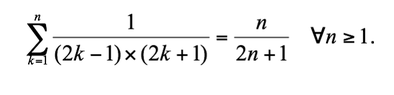# Prove by mathematical induction Σ(1/[(2k-1)(2k+1)]=n/(2n+1)

• MHB
• simcan18
simcan18
I'm in need of assistance for the following attachement.

View attachment 8456

#### Attachments

•Screen Shot 2018-10-05 at 9.11.34 AM.png
4.1 KB · Views: 25
Last edited by a moderator:

I know this is a cliche, but you should really show your work (also in http://mathhelpboards.com/pre-calculus-21/prove-induction-25021.html), not just because it makes others happy, but mostly because it is not useful, nor fun, to be spoon-fed an induction proof.

You are probably aware of the general structure of this proof technique, but in what is perhaps its most elementary form it looks like this. You want to prove a statement for all $n \ge n_0$ where $n_0$ is an integer:

step 0. You verify the base case, i.e. you verify the statement holds for $n = n_0$. Often, but not always, $n_0 = 0$ or $n_0 = 1$.
step 1. You verify: If the statement holds for all $n = n_0,\ldots,m$ where $m \ge n_0$ is a certain integer, then the statement also holds for $n = m + 1$.

Step 0 cannot be omitted, although it is often tempting to do so.

Now, in these two threads, why don't you start by verifying step 0. If that checks out, then formulate the induction hypotheses. (This hypothesis is the part between if and then in step 1.) Finally, take all the time that is required to perform the induction step and do not give up on it too easily.

Last edited:
simcan18
Thanks, but I'm unsure of how to get started with the problem as I don't understand which is the reason for no work being shown.

simcan18
So, do I start like this
n=1
1 / (2x1-1) x(2x1+1) = 1/ 2(1) + 1 = 1/3, so both equations are true for n=1, as it is 1/3

Gold Member
MHB
Okay, once you've shown the base case is true, you want to decide what your inductive step is going to be, given the hypothesis $$P_n$$:

$$\displaystyle \sum_{k=1}^n\left(\frac{1}{(2k-1)(2k+1)}\right)=\frac{n}{2n+1}$$

I see that in order for the sum on the LHS to go up to $$n+1$$, we need to add:

$$\displaystyle \frac{1}{(2(n+1)-1)(2(n+1)+1)}=\frac{1}{(2n+1)(2n+3)}$$

And this gives us:

$$\displaystyle \sum_{k=1}^n\left(\frac{1}{(2k-1)(2k+1)}\right)+\frac{1}{(2(n+1)-1)(2(n+1)+1)}=\frac{n}{2n+1}+\frac{1}{(2n+1)(2n+3)}$$

Or:

$$\displaystyle \sum_{k=1}^{n+1}\left(\frac{1}{(2k-1)(2k+1)}\right)=\frac{n}{2n+1}+\frac{1}{(2n+1)(2n+3)}$$

Can you show that the RHS is:

$$\displaystyle \frac{n+1}{2(n+1)+1}$$ ?

If you can, then you will have derived $$P_{n+1}$$ from $$P_n$$ thereby completing the proof by induction. :)

simcan18
Ok. I'll work on this some more to see if I can get it.

Thanks